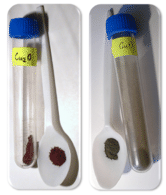7.7: Stock System Naming

In describing many technological items, it's not enough to simply say what brand or model we have. We talk about details such as how much horsepower is "under the hood" for a car or how fast the chip is for our computer. Even a simple device like an mp3 player has more than one size. We can get an 8MB player, or a 16MB player. Designation of the item often is incomplete without other information as to its capabilities.

Transition metals have more than one possibility for ion formation. In order to name these compounds correctly, we need to be able to indicate which ion is involved in any given compound.

Naming Compounds Using the Stock System

Naming compounds that involve transition metal cations necessitates that use of the Stock system. Consider the binary ionic compound $$\ce{FeCl_3}$$. To simply name this compound iron chloride would be incomplete because iron is capable of forming two ions with different charges. The name of any iron-containing compound must reflect which iron ion is the compound. In this case, the subscript in the formula indicates that there are three chloride ions, each with a $$1-$$ charge. Therefore, the charge of the single iron ion must be $$3+$$. The correct name of $$\ce{FeCl_3}$$ is iron (III) chloride, with the cation charge written as the Roman numeral. Here are several other examples.

Formula Name
Table $$\PageIndex{1}$$
$$\ce{Cu_2O}$$ copper (I) oxide
$$\ce{CuO}$$ copper (II) oxide
$$\ce{SnO_2}$$ tin (IV) oxide

The first two examples are both oxides of copper (shown in the figure below). The ratio of copper ions to oxide ions determines the name. Since the oxide ion is $$\ce{O^{2-}}$$, the charges of the copper ion must be $$1+$$ in the first formula and $$2+$$ in the second formula. In the third formula, there is one tin ion for every two oxide ions. This means that the tin must carry a $$4+$$ charge, making the name tin (IV) oxide.Figure $$\PageIndex{1}$$: Copper (II) oxide, a red solid, and copper (II) oxide, a black solid, are different compounds because of the charge of the copper ion.

Summary

• The Stock system allows the specification of transition metal ionic charge when naming ionic compounds.
• Roman numerals are used to indicate the amount of positive charge on the cation.

Contributors

• CK-12 Foundation by Sharon Bewick, Richard Parsons, Therese Forsythe, Shonna Robinson, and Jean Dupon.